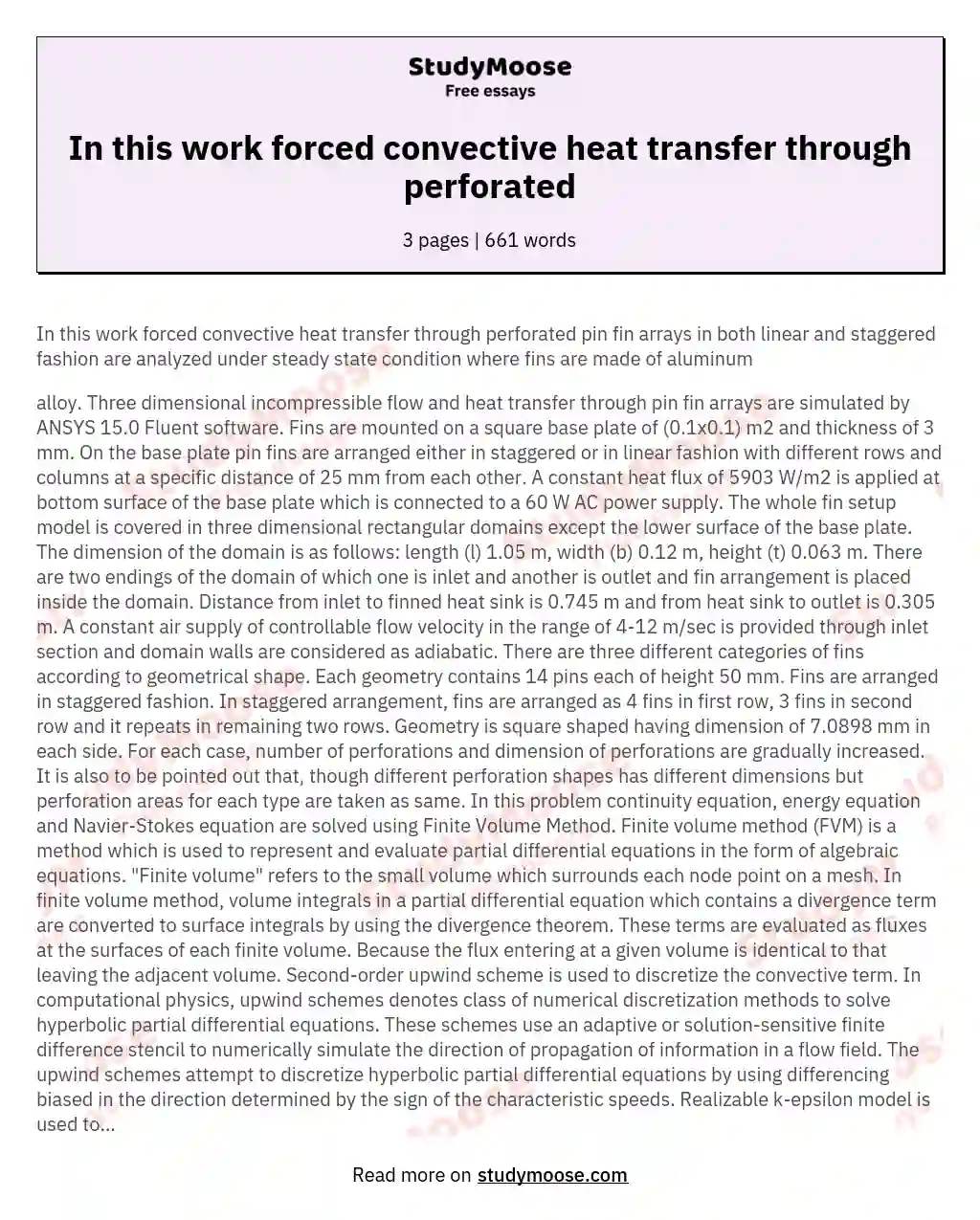In this work forced convective heat transfer through perforated

In this work forced convective heat transfer through perforated pin fin arrays in both linear and staggered fashion are analyzed under steady state condition where fins are made of aluminum

alloy. Three dimensional incompressible flow and heat transfer through pin fin arrays are simulated by ANSYS 15.0 Fluent software. Fins are mounted on a square base plate of (0.1×0.1) m2 and thickness of 3 mm. On the base plate pin fins are arranged either in staggered or in linear fashion with different rows and columns at a specific distance of 25 mm from each other.

A constant heat flux of 5903 W/m2 is applied at bottom surface of the base plate which is connected to a 60 W AC power supply. The whole fin setup model is covered in three dimensional rectangular domains except the lower surface of the base plate. The dimension of the domain is as follows: length (l) 1.05 m, width (b) 0.12 m, height (t) 0.063 m. There are two endings of the domain of which one is inlet and another is outlet and fin arrangement is placed inside the domain.

Get quality help nowRhizManVerified writer

Proficient in: Chemistry4.9 (247)

“ Rhizman is absolutely amazing at what he does . I highly recommend him if you need an assignment done ”+84 relevant experts are online

Distance from inlet to finned heat sink is 0.745 m and from heat sink to outlet is 0.305 m. A constant air supply of controllable flow velocity in the range of 4-12 m/sec is provided through inlet section and domain walls are considered as adiabatic. There are three different categories of fins according to geometrical shape. Each geometry contains 14 pins each of height 50 mm. Fins are arranged in staggered fashion. In staggered arrangement, fins are arranged as 4 fins in first row, 3 fins in second row and it repeats in remaining two rows.

Get to Know The Price Estimate For Your Paper
Topic
Number of pages
Email Invalid email

You won’t be charged yet!

Geometry is square shaped having dimension of 7.0898 mm in each side. For each case, number of perforations and dimension of perforations are gradually increased. It is also to be pointed out that, though different perforation shapes has different dimensions but perforation areas for each type are taken as same. In this problem continuity equation, energy equation and Navier-Stokes equation are solved using Finite Volume Method. Finite volume method (FVM) is a method which is used to represent and evaluate partial differential equations in the form of algebraic equations. “Finite volume” refers to the small volume which surrounds each node point on a mesh. In finite volume method, volume integrals in a partial differential equation which contains a divergence term are converted to surface integrals by using the divergence theorem. These terms are evaluated as fluxes at the surfaces of each finite volume. Because the flux entering at a given volume is identical to that leaving the adjacent volume. Second-order upwind scheme is used to discretize the convective term. In computational physics, upwind schemes denotes class of numerical discretization methods to solve hyperbolic partial differential equations. These schemes use an adaptive or solution-sensitive finite difference stencil to numerically simulate the direction of propagation of information in a flow field. The upwind schemes attempt to discretize hyperbolic partial differential equations by using differencing biased in the direction determined by the sign of the characteristic speeds. Realizable k-epsilon model is used to simulate the turbulence together with the standard wall function and velocity and pressure coupling is achieved by SIMPLEC algorithm. The SIMPLEC (Semi-Implicit Method for Pressure Linked Equations-Consistent) algorithm; which a modified form of SIMPLE algorithm; a commonly used numerical procedure in Computational Fluid Dynamics to solve the Navier-Stokes equations. This algorithm follows the same steps like SIMPLE algorithm but with a little variation that the momentum equations are manipulated which allows SIMPLEC velocity correction equations to omit terms that are less significant than those omitted in SIMPLE. Basically, it tries to prevent the effects of dropping velocity neighbor correction terms. Boundary condition is provided in following way: (i) A constant heat flux of 5903 W/m2 at fin base (ii) inlet velocity varies from 4 m/sec to 12 m/sec where turbulence intensity is 10% and hydraulic diameter is 0.067 m. The thermal energy is transferred at the base of fin array and being dissipated through forced convection.